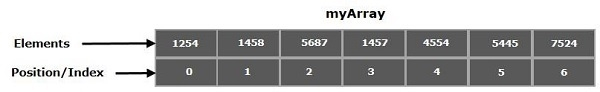# How do you print the content of an array in Java?

In general, arrays are the containers that store multiple variables of the same datatype. These are of fixed size and the size is determined at the time of creation. Each element in an array is positioned by a number starting from 0.You can access the elements of an array using name and position as −

System.out.println(myArray);
//Which is 1457

## Creating an array in Java

In Java, arrays are treated as referenced types you can create an array using the new keyword similar to objects and populate it using the indices as −

int myArray[] = new int;
myArray = 1254;
myArray = 1458;
myArray = 5687;
myArray = 1457;
myArray = 4554;
myArray = 5445;
myArray = 7524;

Or, you can directly assign values with in flower braces separating them with commas (,) as −

int myArray = { 1254, 1458, 5687, 1457, 4554, 5445, 7524};

## Printing the contents of an array

You can print the contents of an array

• element by element − You can access the 1st element of the array as myArray and the second element as myArray and so on using these notation you can the array contents element by element as −
public class PrintingArray {
public static void main(String args[]) {
//Creating an array
int myArray[] = new int;
//Populating the array
myArray = 1254;
myArray = 1458;
myArray = 5687;
myArray = 1457;
myArray = 4554;
myArray = 5445;
myArray = 7524;
//Printing Contents one by one
System.out.println("Contents of the array: ");
System.out.println(myArray);
System.out.println(myArray);
System.out.println(myArray);
System.out.println(myArray);
System.out.println(myArray);
System.out.println(myArray);
System.out.println(myArray);
}
}

## Output

Contents of the array:
1254
1458
5687
1457
4554
5445
7524
• Using the for loop − Instead on printing element by element, you can iterate the index using for loop starting from 0 to length of the array (ArrayName.length) and print element at each index.
public class PrintingArray {
public static void main(String args[]) {
//Creating an array
int myArray[] = new int;
//Populating the array
myArray = 1254;
myArray = 1458;
myArray = 5687;
myArray = 1457;
myArray = 4554;
myArray = 5445;
myArray = 7524;
//Printing Contents using for loop
System.out.println("Contents of the array: ");
for(int i=0; i<myArray.length; i++) {
System.out.println(myArray[i]);
}
}
}

## Output

Contents of the array:
1254
1458
5687
1457
4554
5445
7524
• Using the arrays class - The Arrays class of the java.util package provides a method named toString() it accepts an array (of all types) and prints the contents of the given array.
import java.util.Arrays;
public class PrintingArray {
public static void main(String args[]) {
//Creating an array
int myArray[] = new int;
//Populating the array
myArray = 1254;
myArray = 1458;
myArray = 5687;
myArray = 1457;
myArray = 4554;
myArray = 5445;
myArray = 7524;
//Printing Contents using for loop
System.out.println("Contents of the array: ");
System.out.println(Arrays.toString(myArray));
}
}

## Output

Contents of the array:
[1254, 1458, 5687, 1457, 4554, 5445, 7524]